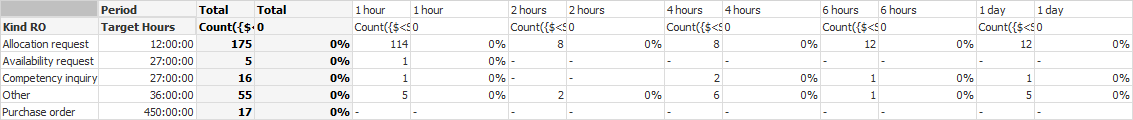# QlikView App Development

Discussion Board for collaboration related to QlikView App Development.

HighlightedNot applicable

## Calculating % of Total

Hi!

I have a new problem. This is my table:My first expressiong is : Count({\$<State={'Processed','Cancelled'},Failed={'Closed','Failed'}>} RORequest)

I need to find the % of Total request for all the Hours using the condition of the first expession.

Or may be I can find the % using the column total? but how can i use the name of thi column in my expression?

Tags (5)
1 Solution

Accepted SolutionsMVP

## Re: Calculating % of Total

Count(TOTAL <[Kind RO]> {\$<State={'Processed','Cancelled'},Failed={'Closed','Failed'}>} RORequest)

9 RepliesMVP

## Re: Calculating % of Total

Try this:

=Count({\$<State={'Processed','Cancelled'},Failed={'Closed','Failed'}>} RORequest)/Count(TOTAL {\$<State={'Processed','Cancelled'},Failed={'Closed','Failed'}>} RORequest)

Best,

SunnyNot applicable

## Re: Calculating % of Total

It's not working, it gives me the total number of all the requests. But I need the total quantity of requests according to the dimension KIND ROMVP

## Re: Calculating % of Total

Not sure what would be the difference between total number and total quantity? In the above image you posted can you point out what the total number vs. total quantity is?

Best,

SunnyMVP

## Re: Calculating % of Total

Hi,

Try with ALL or 1

like,

Count({\$<State={'Processed','Cancelled'},Failed={'Closed','Failed'}>} RORequest)/Count({1<State={'Processed','Cancelled'},Failed={'Closed','Failed'}>} RORequest)

or

Count({\$<State={'Processed','Cancelled'},Failed={'Closed','Failed'}>} RORequest)/Count(TOTAL {<State={'Processed','Cancelled'},Failed={'Closed','Failed'}>} RORequest)

Regards

Great dreamer's dreams never fulfilled, they are always transcended.Not applicable

## Re: Calculating % of Total

I mean that the result of this formula is 268, that is the total quantity of all the requests, and what I need is the total quantity of requests of each Kind RO, like Allocation requests has 175 ,Availability requests has 5 and so on.

But the column that has this numbers is just a partial sum of Pivot Table.MVP

## Re: Calculating % of Total

Give this a try:

=Count({\$<State={'Processed','Cancelled'},Failed={'Closed','Failed'}>} RORequest)/Count(TOTAL <KIND RO> {\$<State={'Processed','Cancelled'},Failed={'Closed','Failed'}>} RORequest)Not applicable

## Re: Calculating % of Total

Hi Max,

I think i havent written all the requirements properly. I need total quantity of each KIND RO.MVP

## Re: Calculating % of Total

Count(TOTAL <[Kind RO]> {\$<State={'Processed','Cancelled'},Failed={'Closed','Failed'}>} RORequest)Not applicable

## Re: Calculating % of Total

That works perfectly!!! Thanks!!!